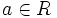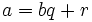# Element of minimum norm among non-units in Euclidean ring is a universal side divisor

## Statement

Suppose$R$ is a commutative unital ring and$N$ is a Euclidean norm on$R$ -- in particular,$R$ is a Euclidean ring. Suppose$b$ is a nonzero element of$R$ that is not a unit, and such that$N(b) \le N(r)$ for all nonzero non-units$r$ of$R$. Then,$b$ is a universal side divisor in$R$.

## Definitions used

### Euclidean norm

Further information: Euclidean norm

### Universal side divisor

Further information: Universal side divisor

A nonzero non-unit$b$ in a commutative unital ring$R$ is termed a universal side divisor in$R$ if for any$a \in R$, either$b | a$ or there exists a unit$r \in R$ such that$b | a - r$.

## Proof

Given: A commutative unital ring$R$ with a Euclidean norm$N$. A nonzero non-unit element$b$ of$R$ such that$N(b) \le N(r)$ for all nonzero non-units$r$ in$R$.

To prove:$b$ is a universal side divisor in$R$.

Proof: Pick any$a \in R$. Then, by the Euclidean algorithm, we can write:$a = bq + r$

where either$r = 0$ or$N(r) < N(b)$. If$r = 0$,$b | a$, and we are done. Otherwise,$N(r) < N(b)$. By assumption,$b$ has smallest norm among the non-units, so$r$ must be a unit, hence$bq = a - r$, so$b | a - r$ for a unit$r$, and we are done.